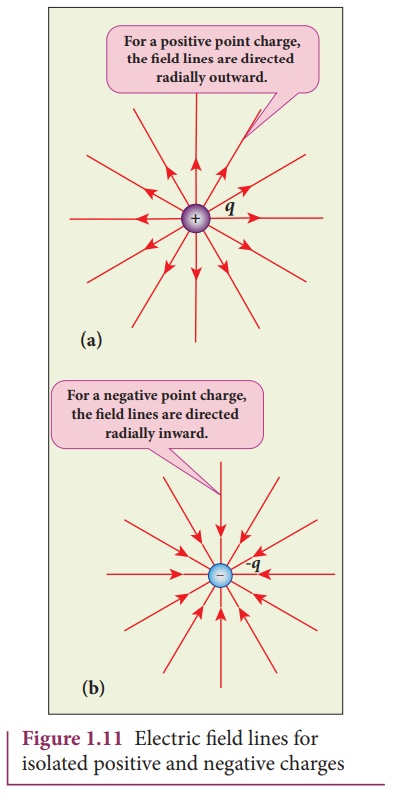Home | | Physics 12th Std | Electric field lines

# Electric field lines

Electric field vectors are visualized by the concept of electric field lines.

Electric field lines

Electric field vectors are visualized by the concept of electric field lines. They form a set of continuous lines which are the visual representation of the electric field in some region of space. The following rules are followed while drawing electric field lines for charges.

The electric field lines start from a positive charge and end at negative charges or at infinity. For a positive point charge the electric field lines point radially outward and for a negative point charge, the electric field lines point radially inward. These are shown in Figure 1.11 (a) and (b).Note that for an isolated positive point charge the electric field line starts from the charge and ends only at infinity. For an isolated negative point charge the electric field lines start at infinity and end at the negative charge.

·        The electric field vector at a point in space is tangential to the electric field line at that point. This is shown in Figure 1.12·        The electric field lines are denser (more closer) in a region where the electric field has larger magnitude and less dense in a region where the electric field is of smaller magnitude. In other words, the number of lines passing through a given surface area perpendicular to the lines is proportional to the magnitude of the electric field in that region. This is shown in Figure1.13Figure 1.13 shows electric field lines from a positive point charge. The magnitude of the electric field for a point charge decreases as the distance increasesSo the electric field has greater magnitude at the surface A than at B. Therefore, the number of lines crossing the surface A is greater than the number of lines crossing the surface B. Note that at surface B the electric field lines are farther apart compared to the electric field lines at the surface A.

·        No two electric field lines intersect each other. If two lines cross at a point, then there will be two different electric field vectors at the same point, as shown in Figure 1.14.As a consequence, if some charge is placed in the intersection point, then it has to move in two different directions at the same time, which is physically impossible. Hence, electric field lines do not intersect.

·        The number of electric field lines that emanate from the positive charge or end at a negative charge is directly proportional to the magnitude of the charges.

For example in the Figure 1.15, the electric field lines are drawn for charges +q and -2q. Note that the number of field lines emanating from +q is 8 and the number of field lines ending at -2q is 16. Since the magnitude of the second charge is twice that of the first charge, the number of field lines drawn for -2q is twice in number than that for charge +q.### EXAMPLE 1.9

The following pictures depict electric field lines for various charge configurations.(i) In figure (a) identify the signs of two charges and find the ratio |q1 / q2|

(ii) In figure (b), calculate the ratio of two positive charges and identify the strength of the electric field at three points A, B, and C

(iii) Figure (c) represents the electric field lines for three charges. If q2 = -20 nC, then calculate the values of q1 and q3

### Solution

(i) The electric field lines start at q2 and end at q1. In figure (a), q2 is positive and q1 is negative. The number of lines starting from q2 is 18 and number of the lines ending at q1 is 6. So q2 has greater magnitude. The ratio of |q1/ q2| = N1/ N2 = 6/18 = 1/3 . It implies that |q2| = 3|q1|

(ii) In figure (b), the number of field lines emanating from both positive charges are equal (N=18). So the charges are equal. At point A, the electric field lines are denser compared to the lines at point B. So the electric field at point A is greater in magnitude compared to the field at point B. Further, no electric field line passes through C, which implies that the resultant electric field at C due to these two charges is zero.

(iii) In the figure (c), the electric field lines start at q1 and q3 and end at q2. This implies that q1 and q3 are positive charges. The ratio of the number of field lines is | q1 / q2 | = 8/16 = | q3 / q2 | = 1/2  , implying that q1and q3are half of the magnitude of q2. So q1 = q3 = +10 nC.

Study Material, Lecturing Notes, Assignment, Reference, Wiki description explanation, brief detail
12th Physics : Electrostatics : Electric field lines |